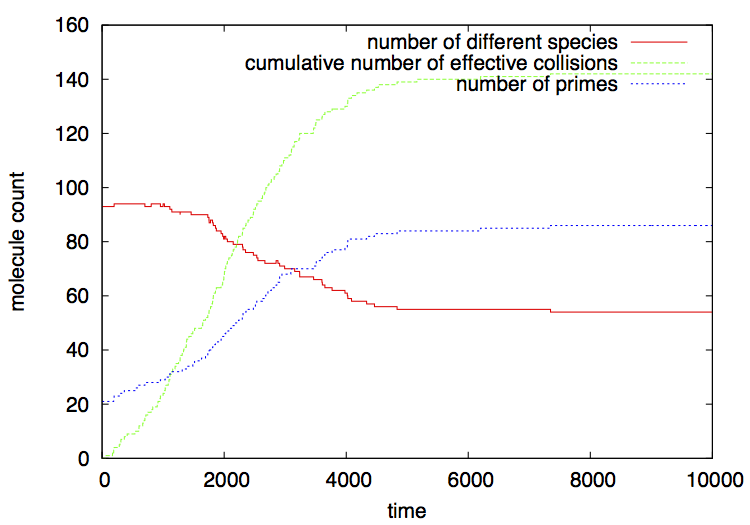[top] [up] [previous] [next]PyCellChemistry: The Number Chemistry Example

The Number Chemistry is a simple example of a constructive AC. Setting up a constructive chemistry is typically more complex than setting up a nonconstructive one. Such initial extra work will be compensated by a much greater flexibility and the open-ended possibilities of molecule types and dynamic behaviors.

Constructive ACs have no fixed recipe for their design, therefore the support from PyCellChemistry can only be very lightweight. Since molecules and reactions may be defined in an implicit way, each constructive chemistry may have its own way to decide when and how reactions take place among molecules, the rules to apply in each case, and the algorithm to simulate their dynamics.

The following modules from PyCellChemistry can typically be used to implement constructive chemistries:

• Multiset (and its variant KeyMultiset): for well-mixed compartments;
• Cell: for multiple hiearchical compartments;
• BinaryStrings: for ACs in which molecules are binary strings;
• HighOrderChem: for ACs where reaction rules are expressed as molecules in a multiset.

The Python implementation

We now turn to the prime number chemistry implemented in NumberChem.py. A partial source code for this chemistry is shown in figure 1. The complete code for this example is a bit larger than the Dimer example, so we skip a few details and focus on the most relevant excerpts of the code.

We start by creating a Multiset mset, and inserting some random numbers in it (lines 4-7 in figure 1). By default the chemistry is initialized with popsize=100 random integers picked from the interval minn=2 to maxn=1000, that are injected into mset. After that, we run niter=10000 iterations of a variant of the following algorithm:

• Extract two random molecules m1 and m2 from the multiset (lines 13-14).
• Lines 15-18 make sure that m2 ≥ m1 by exchanging the values of the two variables if needed.
• Lines 19 and 23 record the collision and its resulting products if any.
• The collision is effective only when m2 ≥ m1 and the division of m2 by m1 has no remainder (line 20). In this case (lines 21-23) the reaction is performed by dividing m2 by m1; the result replaces m2.
• A counter for effective collisions is then incremented (line 22), and the product of the reaction is recorded (line 23).
• Lines 27-28 reinject m1 and m2 into the reactor, oblivious to the fact that one of them may have been modified or not.
The data for plotting are printed in the form of tab-separated columns, using the trace_title (line 10) and trace (lines 11 and 26): trace_title prints the header line with the names of the traced parameters separated by tabs, and trace is invoked at the end of every iteration to print a data line with the values of each parameter separated by tabs. The following parameters are traced: iteration number (column 1), total number of species (column 2), cumulative number of effective collisions up to the present iteration (column 3), total number of molecules representing prime numbers (column 4), and the event that happened in the present iteration (column 5: in case of an elastic collision, only the colliding molecules are shown; in case of an effective collision, the generated products are also shown).

Finally, lines 28-29 create a NumberChem object and invoke the chemistry, running it for a number of iterations (line 27 ensures that the code is executed only when the python script is invoked directly, not when it is included from other scripts).

Running the example

Like most of the chemistry examples provided in PyCellChemistry, there are two ways to run the NumberChem example: either by invoking NumberChem.py directly from Python, or via a shell script.

Invoking the script directly in Python, from a Unix command line shell:

cd pycellchem-2.0/src
python NumberChem.py > numchem.gr

This will produce a tab-separated file numchem.gr containing the traced parameters explained above.

Running it from a shell script

Another way to run this example is to invoke the numchem.sh shell script found in the src/scripts folder:

cd pycellchem-2.0/src
./scripts/dimer.sh

As with the other examples, the script must be involked from the src/ folder, although the script itself is located in the src/scripts folder.

This script invokes Python as before, and plots the output file numchem.gr using gnuplot. A file numchem.eps is produced: it shows how the population of prime numbers increases as the reactions proceed factorizing numbers, until only primes remain. In contrast, the number of different species decreases, as only prime numbers "survive". After no numbers can be broken down, the number of effective collisions reaches a plateau. Figure 2 shows a typical output (remember that your actual output will be different from this one, since this is a stochastic simulation; however qualitatively the results should be very similar). The output can be visualized by simply clicking on it, or from the command line on MacOS with

open numchem.eps

and on Linux with tools such as gv or gimp.

The result is:Figure 2: output of the NumberChem example, as plotted by the numchem.sh script.Last updated: September 19, 2015, by Lidia Yamamoto